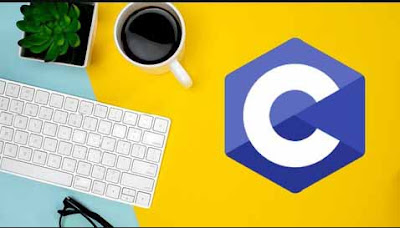# C Programming Examples with Output Part - 5 - Ishwaranand

## ProgramC Programming Examples with Output Part - 5 ~ Image by ©Ishwaranand

### 1) what is the output of the following program? If user inputs the values as AMRAVATI NAGPUR YAVATMAL

void main()
{
char a, b, c;
scanf("%c%c%c", &a, %b, &c);
printf("a=%c b=%c c=%c", a, b, c);
}
Output:
a = A b = M c = R

### 2) what is the output of the following program? - Increment decrement

# include <stdio.h>
void main()
{
int a=7;

printf(""%d"", a++);
printf(""\n%d"", a++ * a++);
printf(""\n%d"", ++a *++a);
}
output:
7
72
132

### 3) what is the output of the following program? Math formulas

#include <stdio.h>
void main()
{
int b=4, c=4, d=2, e=4, i=2,a;

a=b+c\d + e*f;
printf("a=%d",a);

a=(b+c)/d+ e*f;
printf("\na=%d", a);

a= b+c/((d+e)*f);
printf("\na=%d",a);
}
output:
a=16
a=14
a=4